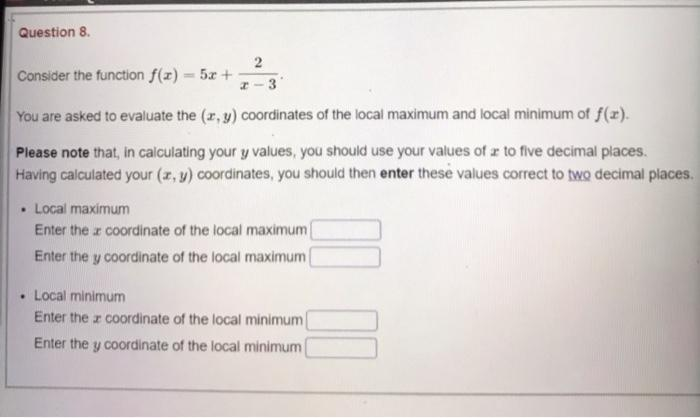### Create an Account

Already have account?

### Forgot Your Password ?

Home / Questions / Question 8 2 Consider the function f(x) = 5x + -3 You are asked to evaluate the (x, y) coo...

# Question 8 2 Consider the function f(x) = 5x + -3 You are asked to evaluate the (x, y) coordinates of the local maximum and local minimum of f(x). Please note that, in calculating your y values, you s

Question 8 2 Consider the function f(x) = 5x + -3 You are asked to evaluate the (x, y) coordinates of the local maximum and local minimum of f(x). Please note that, in calculating your y values, you should use your values of x to five decimal places. Having calculated your (x, y) coordinates, you should then enter these values correct to two decimal places. • Local maximum Enter the x coordinate of the local maximum Enter the y coordinate of the local maximum • Local minimum Enter the x coordinate of the local minimum Enter the y coordinate of the local minimumApr 15 2021 View more View Less

#### Answer (Solved)Subscribe To Get Solution